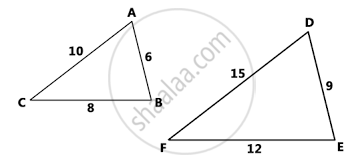# Similar Figures

## Definition

Two figures that have the same shape are said to be similar. When two figures are similar, the ratios of the lengths of their corresponding sides are equal. To determine if the triangles below are similar, compare their corresponding sides.## Notes

How will be state that the above figures are similar? ∆ABC ∼ ∆DEF are similar if
1) The corresponding angles are same in measurement i.e ∠A≅∠D, ∠B≅∠E ,∠C≅∠F
AND 2) The corresponding sides are of same ratios i.e "AB"/"DE"="BC"/"EF"= "AC"/"DF"
Thus the figures above are Similar Figures
Also we can say that if ∠A≅∠D, ∠C≅∠F, ∠B≅∠E then "AC"/"DF"= "CB"/"FE"= "BA"/"ED"
OR if "AC"/"DF"= "CB"/"FE"= "BA"/"ED" then ∠A≅∠D, ∠C≅∠F, ∠B≅∠E
This is further explained in later concepts with help of theorem.

If you would like to contribute notes or other learning material, please submit them using the button below.

### Shaalaa.com

Triangles part 1 (Similar Figures) [00:11:01]
S
0%